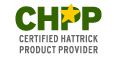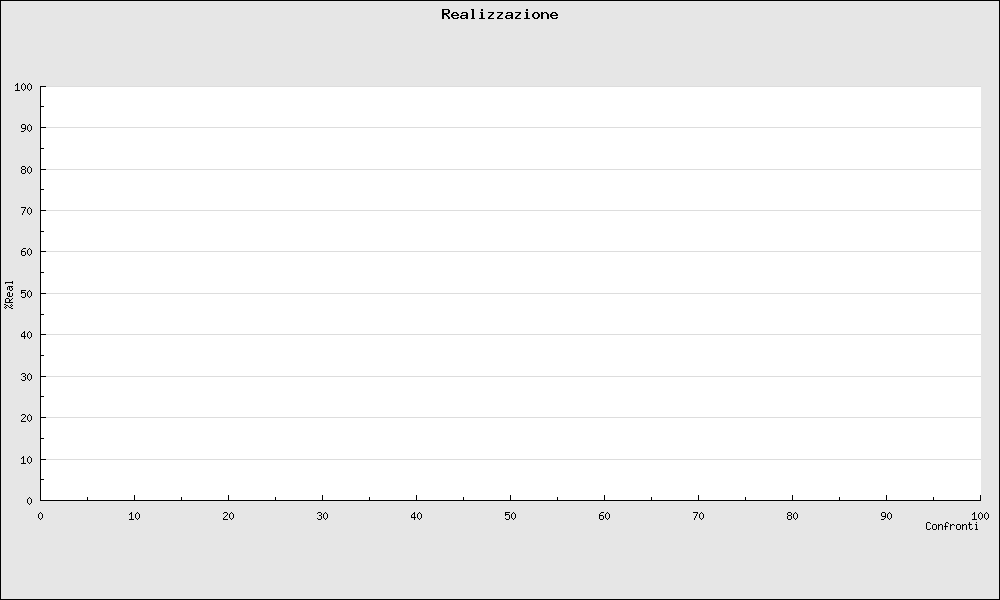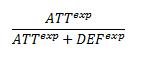'; \$table.= '"; \$table.="

#Hattrick Match Analysis28 May 2020 Pour toute question, contactez taised sur HattrickCustom Search

"; \$table.= ''; \$table.= ''; for (\$i=1;\$i<=\$t;\$i++) { \$z=\$i-1; \$table.= ''; \$table.= ""; \$table.= ""; \$table.= ""; \$table.= ""; \$table.= ""; \$table.= ""; \$table.= ""; \$table.= ""; \$table.= ''; } \$table.= '
 '.getLangString(\$langDOM, "realGraphTable1").' '.getLangString(\$langDOM, "realGraphTable2").' '.getLangString(\$langDOM, "realGraphTable3").' '.getLangString(\$langDOM, "realGraphTable4").' '.getLangString(\$langDOM, "realGraphTable5").' '.getLangString(\$langDOM, "realGraphTable6").' '.getLangString(\$langDOM, "realGraphTable7").' '.getLangString(\$langDOM, "realGraphTable8").' \$x2defdata[\$z] \$xdefdata[\$z] \$ydefdata[\$z] \$ndefdata[\$z] ". round((\$ydefdata[\$z]/100-1.96*(sqrt(((\$ydefdata[\$z]/100)*(1-(\$ydefdata[\$z]/100)))/\$ndefdata[\$z])))*100,2) ." ". round((\$ydefdata[\$z]/100+1.96*(sqrt(((\$ydefdata[\$z]/100)*(1-(\$ydefdata[\$z]/100)))/\$ndefdata[\$z])))*100,2) ." \$M[\$z] ".(round(sqrt((\$xdefdata[\$z]/100)*(1-(\$xdefdata[\$z]/100))),2))."
'; \$table.= '
'; //Legenda \$table.="".getLangString(\$langDOM, "legend")."
"; \$table.=""; \$table.=""; \$table.=""; \$table.=""; \$table.=""; \$table.=""; \$table.=""; \$table.=""; \$table.="
 "; \$table.="".getLangString(\$langDOM, "realGraphTable1")." : ".getLangString(\$langDOM, "realGraphTable1Text")." ".getLangString(\$langDOM, "realGraphTable2")." : ".getLangString(\$langDOM, "realGraphTable2Text")." ".getLangString(\$langDOM, "realGraphTable3")." : ".getLangString(\$langDOM, "realGraphTable3Text")." ".getLangString(\$langDOM, "realGraphTable4")." : ".getLangString(\$langDOM, "realGraphTable4Text")." ".getLangString(\$langDOM, "realGraphTable5")." : ".getLangString(\$langDOM, "realGraphTable5Text")." ".getLangString(\$langDOM, "realGraphTable6")." : ".getLangString(\$langDOM, "realGraphTable6Text")." ".getLangString(\$langDOM, "realGraphTable7")." : ".getLangString(\$langDOM, "realGraphTable7Text")." ".getLangString(\$langDOM, "realGraphTable8")." : ".getLangString(\$langDOM, "realGraphTable8Text")."
"; \$table.="
"; return \$table; } function display_graph_ajax(\$lang ,\$maxint,\$mindati,\$min,\$max,\$exp) { //lang \$langDOM=new DomDocument(); \$langDOM->load('locale/lang.'.\$lang.'.xml'); //timer \$pntTime=new executionScript(); \$pntTime->start(); \$values= array(); \$values['maxint']=\$maxint; \$values['mindati']=\$mindati; \$values['min']=\$min; \$values['max']=\$max; \$values['exp']=\$exp; \$graphGen=new GraphGen; \$results=\$graphGen->makeGraph(\$myconn, \$values); \$x2defdata=\$results['x2defdata']; \$xdefdata=\$results['xdefdata']; \$ydefdata=\$results['ydefdata']; \$ndefdata=\$results['ndefdata']; \$M=\$results['M']; \$n=\$results['n']; //codifica per GET \$coded_array = base64_encode(serialize(\$xdefdata)); \$encoded = strtr(base64_encode(addslashes(gzcompress(serialize(\$coded_array),9))), '+/=', '-_,'); \$coded_array = base64_encode(serialize(\$ydefdata)); \$yencoded = strtr(base64_encode(addslashes(gzcompress(serialize(\$coded_array),9))), '+/=', '-_,'); \$coded_array = base64_encode(serialize(\$ndefdata)); \$nencoded = strtr(base64_encode(addslashes(gzcompress(serialize(\$coded_array),9))), '+/=', '-_,'); //PREPARAZione link \$imgraph= ""; //\$risultato='displayGraph(\$xdefdata, \$ydefdata, \$ndefdata).'?rand='.rand()%100 .' id="graphimg" border=0 align=top >

'; \$risultato='
'.\$imgraph.'

'; \$pntTime->stop(); \$risultato.=''.getLangString(\$langDOM, "realGraphResult1").': '.\$mindati.', '.getLangString(\$langDOM, "realGraphResult2").': '.\$maxint.'%, '.getLangString(\$langDOM, "realGraphResult3").' '.\$n.' '.getLangString(\$langDOM, "realGraphResult4").' (ms): '.\$pntTime->getTime().''; \$risultato.='

'; \$tabella=display_table(\$xdefdata, \$ydefdata, \$x2defdata, \$ndefdata, \$M, \$langDOM); return \$risultato.\$tabella; //return array('grafico'=>\$risultato, 'tabella'=>\$tabella); } // Necessary PHPLiveX Codes \$ajax2 = new PHPLiveX(array("display_graph_ajax")); \$ajax2->Run(); ?>

## Realization stats (by GM-HomerJay)

### Introduction

We included only counter attack chances, because they're all reported in the match report, so that we didn't have to care about missed chances not reported.
Graph represent the effective percentage of realization for the CA chances in our database, you've the possibility to change some factor in order to see what will change, click "Generate" in order to have the refreshed graph.
n Y you'll find the effective realization's percentage of the considered chances (goals/tot).
Instead in X you'll find the theorical result of our function, that we suppose to decide the realization probability, this is the general structure:Where:
ATT: Attack rating
DEF: Defence (opponent) rating
EXP: exp, factor decided by you

Factors
 Max width of single interval size="4" maxlength="4" /> Max data for interval size="4" maxlength="4" /> Min rating included size="4" maxlength="4" /> Max rating included size="4" maxlength="4" /> Exponent size="4" maxlength="4" />

### Graph

The Black line represent the main data, the real realization.
The Blue line represent the ideal data, our function will be more accurate if the black line is near this one
The Green and Orange lines represent the upper and lower limit of the confidence interval. This interval is calculated from the data available for each interval and can be defined as the value for which the data is valid. If the data is calculated as an approximation of the binomial function, in this interval is included 95% of real results of the game engine.

### Conversion graph for HO

This is created in order to be comparable with the function that HO use to compare ratings, here you'll find the result for our data.
Function is (ATT-DEF)/DEF#### Zoom on useful interval (up to +50)### Some more explanation

We collected data of matches played after 20 may 2009, since when the conficence effect is visible in ratings
We collected only CA chances, in order to don't care about not reported chances.
We collected data automatically from schedule of italian leagues, so the database is not influenced by a manual reported match. We set some filters on the data in order to be sure that they wouldn't be influenced too much, we excluded matches where there was one of this event verified:
• Confusion
• Substitutions due to injury
• Red cards
• OverConfidence
Other problems unluckily were not solvable with filters and so still caused possible wrong effects:
1. Individual orders and conditional subs

2. Going defensiveCookie policy Developer taised -  french version courtesy of Stephan Cardot (stephan57)